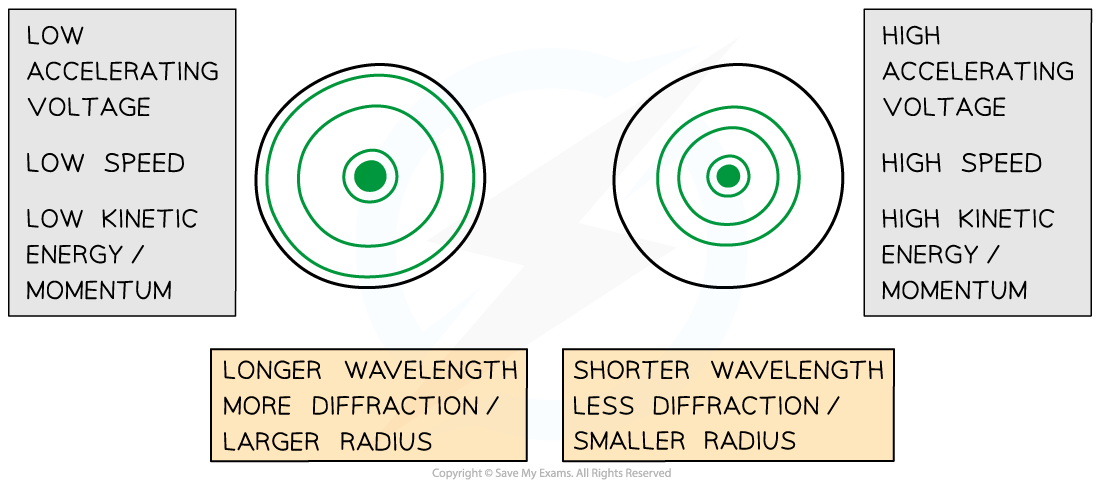# AQA A Level Physics复习笔记2.5.5 Diffraction Effects of Momentum

### Diffraction Effects of Momentum

• When electrons pass through a slit similar in size to themselves, they exhibit a wavelike property( Diffraction), meaning they spread out like a wave passing through a narrow gap
• The regular spacing of atoms in a crystalline solid act as a diffraction grating, scattering the electrons in a predictable manner
• The observed diffraction pattern can be used to deduce the structure of the crystal producing that pattern
• High energy electrons have a shorter wavelength and can therefore be used to look at the size of the nucleus of an atom as opposed to the arrangement of atoms in a crystal
• The de Broglie wavelength tells us about the wave-particle relationship:• Where:
• λ = the de Broglie wavelength (m)
• h = Planck’s Constant (J s)
• m = mass of the electron (kg)
• v = velocity of the electron (m s–1)Comparison of electron diffraction patterns at different values of momentum

#### Momentum

• Momentum is equal to p = mv, so, from de Broglie's equation:
• A smaller momentum will result in a longer wavelength
• A larger momentum will result in a shorter wavelength

#### Kinetic Energy

• If the electron speed / kinetic energy is increased, by increasing the accelerating voltage, then:
• The wavelength of the wave will decrease
• The diffraction rings will appear closer together

• The higher the kinetic energy of the electron, the higher its momentum hence the smaller its wavelength

#### Radius of the Diffraction Pattern

• The radius of the diffraction pattern depends on the wavelength:
• The longer the wavelength, the more the light spreads out hence a larger radius is produced
• The shorter the wavelength, the smaller the radius produced

• Therefore, electrons with smaller momentum will produce a more diffuse diffraction pattern